# BRADLEY UNIVERSITY Department of Electrical and Computer Engineering

• Slides: 53
Download presentationBRADLEY UNIVERSITY Department of Electrical and Computer Engineering Sr. Capstone Project Student: Paul Friend Advisor: Dr. Anakwa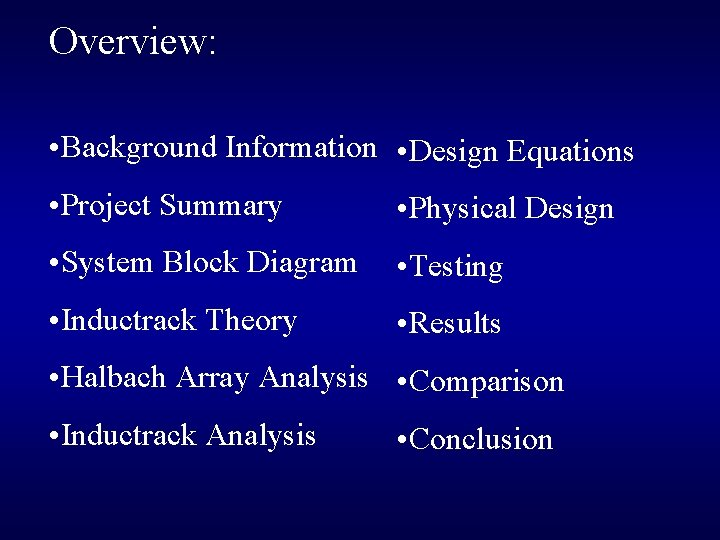Overview: • Background Information • Design Equations • Project Summary • Physical Design • System Block Diagram • Testing • Inductrack Theory • Results • Halbach Array Analysis • Comparison • Inductrack Analysis • ConclusionBackground Information Choice - Inductrack: • Newest method for Maglev trains • Does not require high power for operation • Does not require complex controls for stability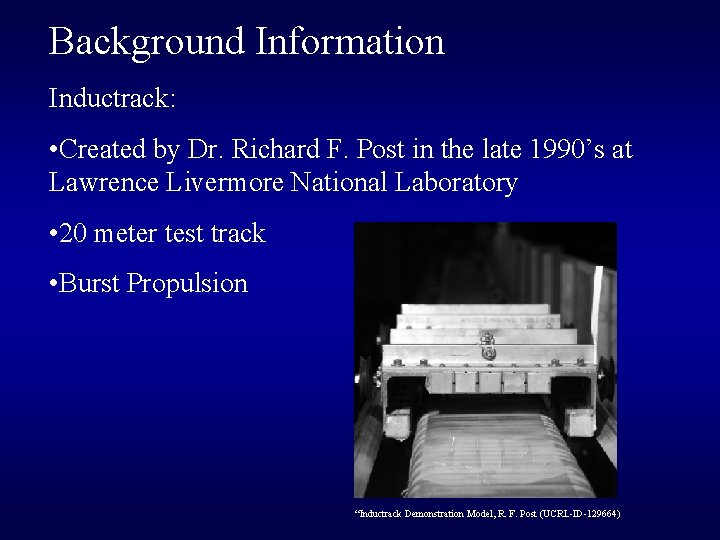Background Information Inductrack: • Created by Dr. Richard F. Post in the late 1990’s at Lawrence Livermore National Laboratory • 20 meter test track • Burst Propulsion “Inductrack Demonstration Model, R. F. Post (UCRL-ID-129664)Background Information Inductrack: • Contracted by NASA for Satellite Launcher • Low-Speed Urban Maglev Program “Maglev on the Development Track for Urban Transportation, LLNLProject Summary • Determine and Understand the Inductrack Theory • Design and Simulate a levitating train utilizing the Inductrack Theory • Build a levitating train and track • Test the Inductrack parameters • If time allows, design and test a propulsion systemSystem Block Diagram High Level: Desired Velocity Maglev System Train Velocity Levitation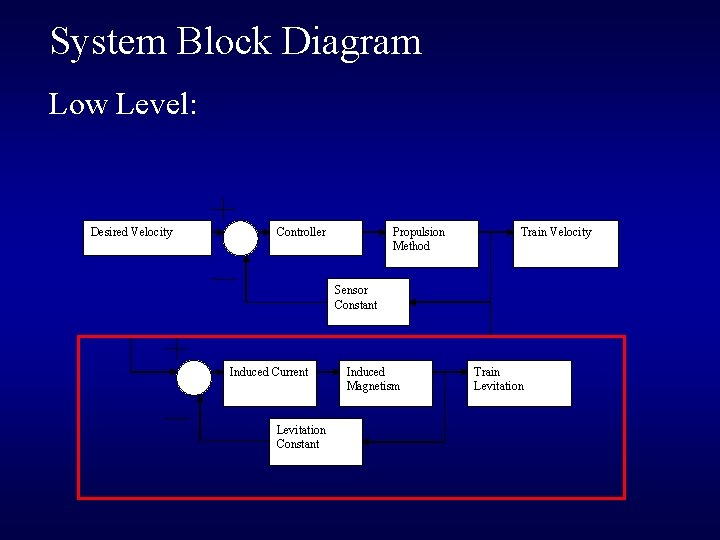System Block Diagram Low Level: Desired Velocity Propulsion Method Controller Train Velocity Sensor Constant Induced Current Levitation Constant Induced Magnetism Train Levitation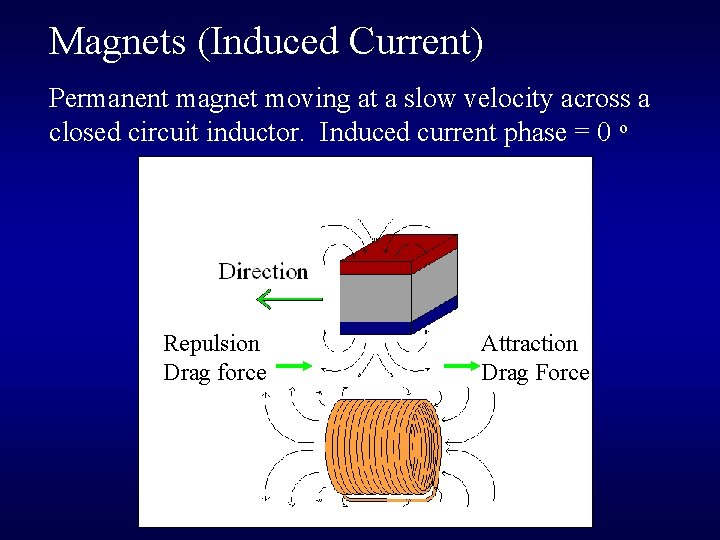Magnets (Induced Current) Permanent magnet moving at a slow velocity across a closed circuit inductor. Induced current phase = 0 o Repulsion Drag force Attraction Drag Force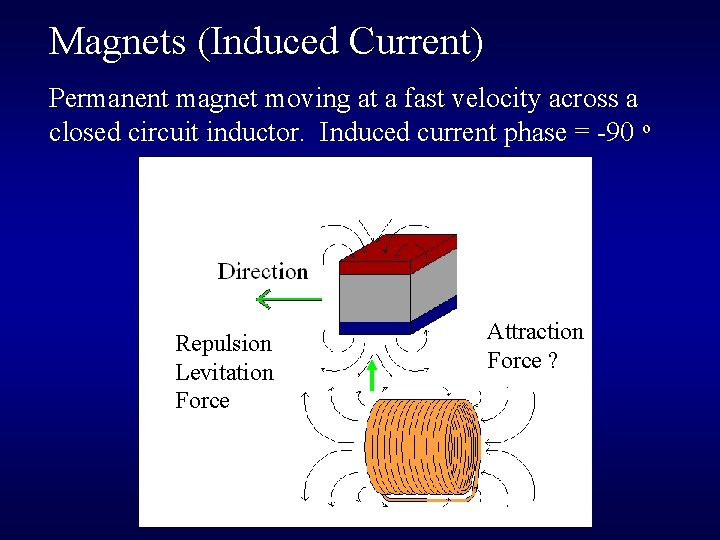Magnets (Induced Current) Permanent magnet moving at a fast velocity across a closed circuit inductor. Induced current phase = -90 o Repulsion Levitation Force Attraction Force ?Halbach Array • Created by Klaus Halbach • Creates a strong, nearly one-sided magnet with a sinusoidal field by directing the magnetic fields.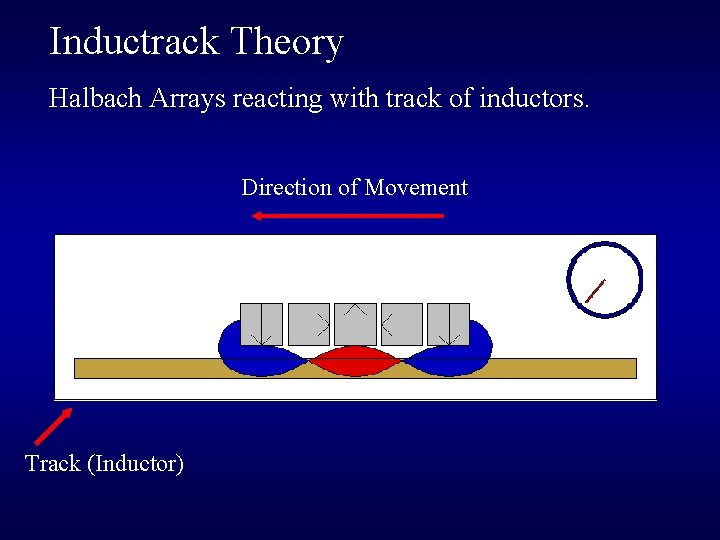Inductrack Theory Halbach Arrays reacting with track of inductors. Direction of Movement Track (Inductor)Inductrack Inductor Physics • Lenz’s Law • Discovered in 1834 • Eddy currents created due to moving magnetic field • (Not guided)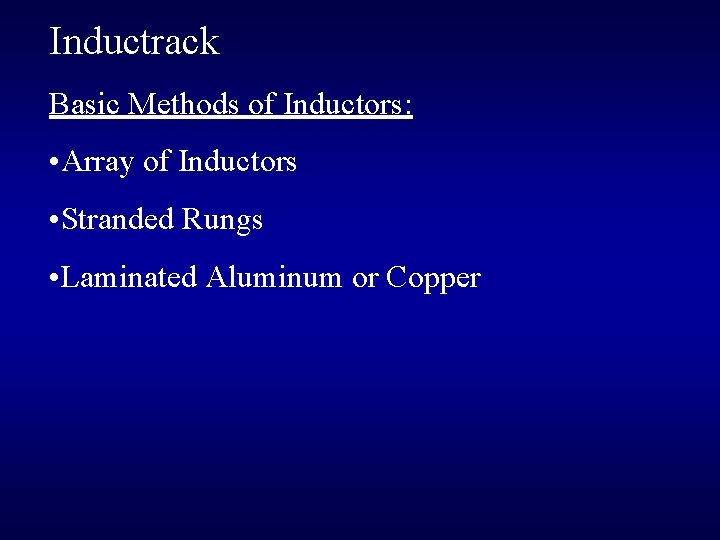Inductrack Basic Methods of Inductors: • Array of Inductors • Stranded Rungs • Laminated Aluminum or Copper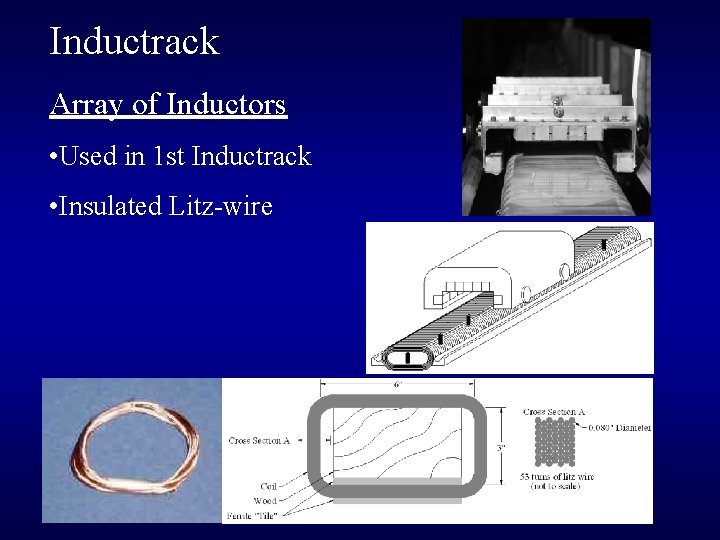Inductrack Array of Inductors • Used in 1 st Inductrack • Insulated Litz-wire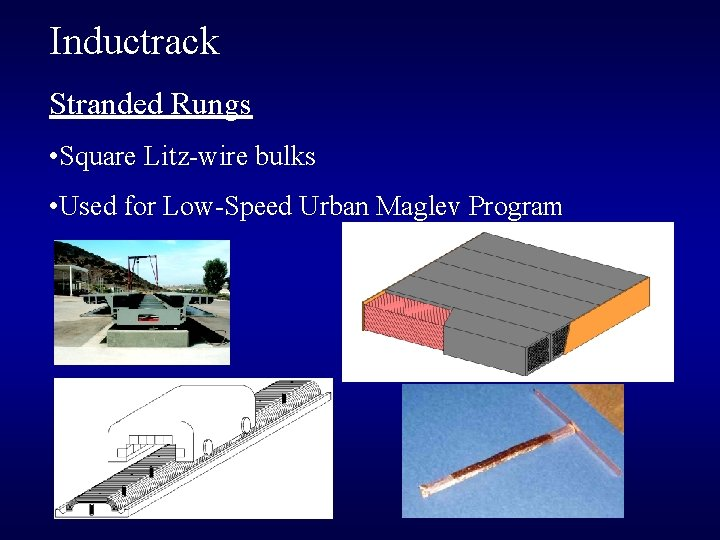Inductrack Stranded Rungs • Square Litz-wire bulks • Used for Low-Speed Urban Maglev Program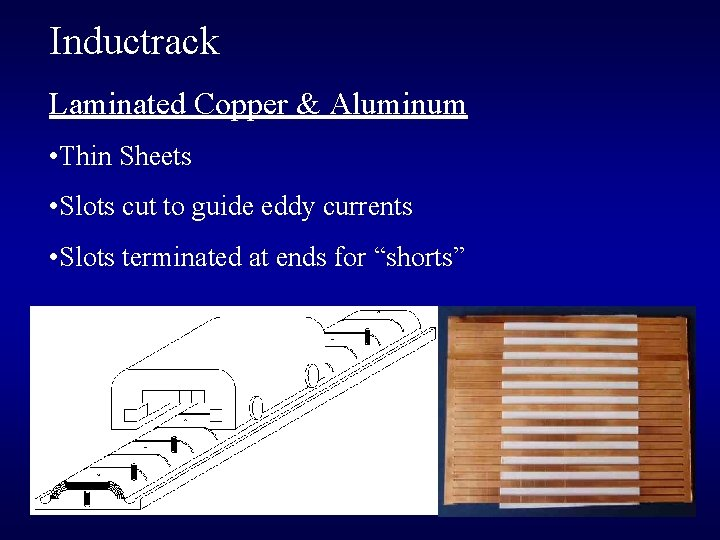Inductrack Laminated Copper & Aluminum • Thin Sheets • Slots cut to guide eddy currents • Slots terminated at ends for “shorts”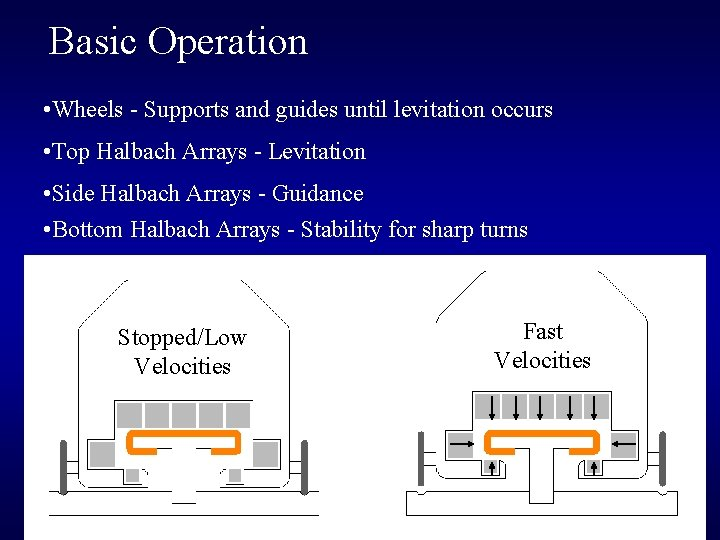Basic Operation • Wheels - Supports and guides until levitation occurs • Top Halbach Arrays - Levitation • Side Halbach Arrays - Guidance • Bottom Halbach Arrays - Stability for sharp turns Stopped/Low Velocities Fast Velocities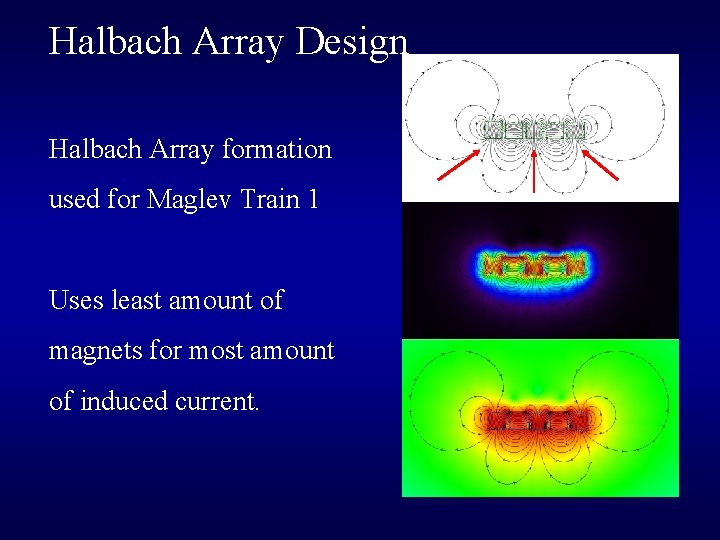Halbach Array Design Halbach Array formation used for Maglev Train 1 Uses least amount of magnets for most amount of induced current.Inductrack Simulations Stopped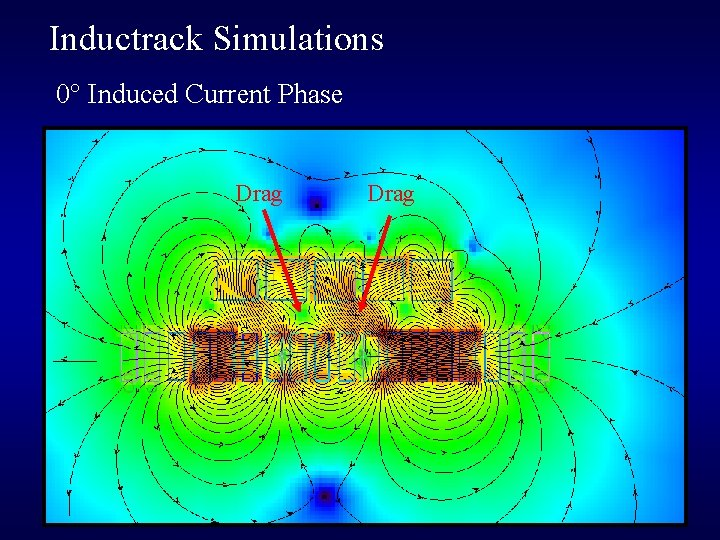Inductrack Simulations 0° Induced Current Phase Drag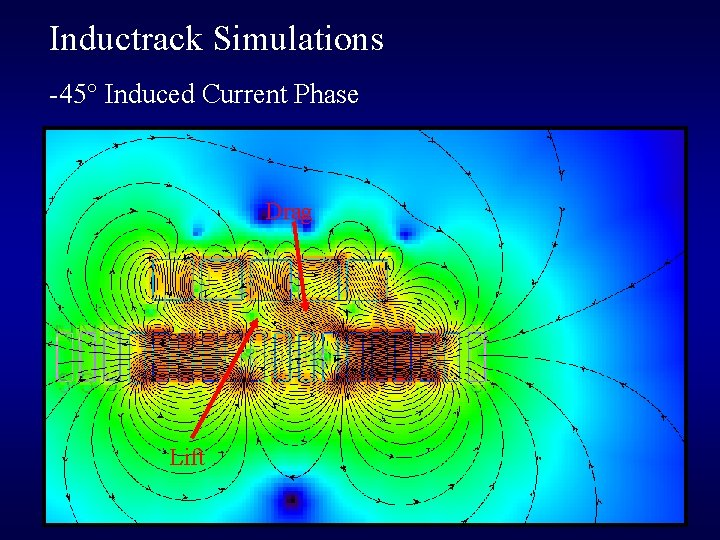Inductrack Simulations -45° Induced Current Phase Drag Lift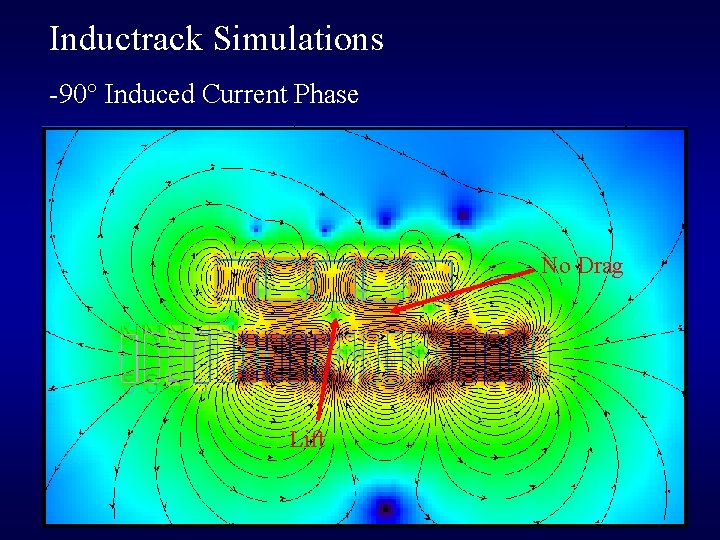Inductrack Simulations -90° Induced Current Phase No Drag LiftCircuit Theory I(s) = (V/L)/(R/L + s) Pole at R/L Note: V increases with velocityDesign Equations (Magnetic Fields) B 0 = Br (1 – e-2πd/λ)[(sin(π/M))/( π/M)] [Tesla] B 0 = 0. 82843 (1/2” Gr. 38 Nd. Fe. B Cube Magnets) Bx = B 0 sin((2π/λ)x) e-(2π/λ) (y 1 – y) [Tesla] By = B 0 cos((2π/λ)x) e-(2π/λ) (y 1 – y) [Tesla]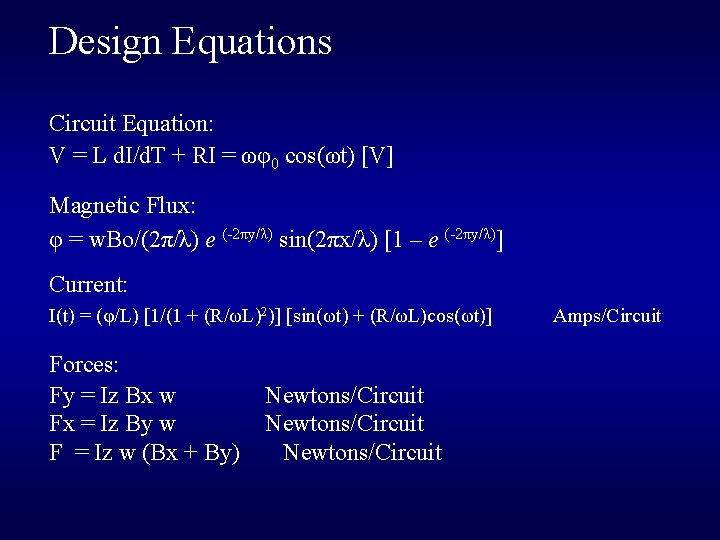Design Equations Circuit Equation: V = L d. I/d. T + RI = ωφ0 cos(ωt) [V] Magnetic Flux: φ = w. Bo/(2π/λ) e (-2πy/λ) sin(2πx/λ) [1 – e (-2πy/λ)] Current: I(t) = (φ/L) [1/(1 + (R/ωL)2)] [sin(ωt) + (R/ωL)cos(ωt)] Forces: Fy = Iz Bx w Fx = Iz By w F = Iz w (Bx + By) Newtons/Circuit Amps/CircuitDesign Equations Forces: Levitation Force: Fy(ω) = levs[Bo 2 w/(4πL dc/λ)] [ 1/(1 + (R/ωL)2)]A e (-4π y/λ) Newtons Fy(s) = levs[Bo 2 w/(4πL dc/λ)] {(L 2 s 2)/[(s - R/L) (s + R/L)]} A e (-4π y/λ) Newtons Drag Force: Fx(ω) = levs[Bo 2 w/(4πL dc/λ)] [ (R/ωL)/(1 + (R/ωL)2)]A e (-4π y/λ) Newtons Fx (s) = levs[Bo 2 w/(4πL dc/λ)] {(RL s)/[(s - R/L) (s + R/L)]} A e (-4π y/λ) Newtons Levitation Force: F (ω) = Fy(ω) + Fx(ω) Newtons F(s) = levs[Bo 2 w/(4πL dc/λ)] [(L 2 s)/(s + R/L)] A e (-4π y/λ) Newtons Lift/Drag = <Fy>/<Fx> = ω L/R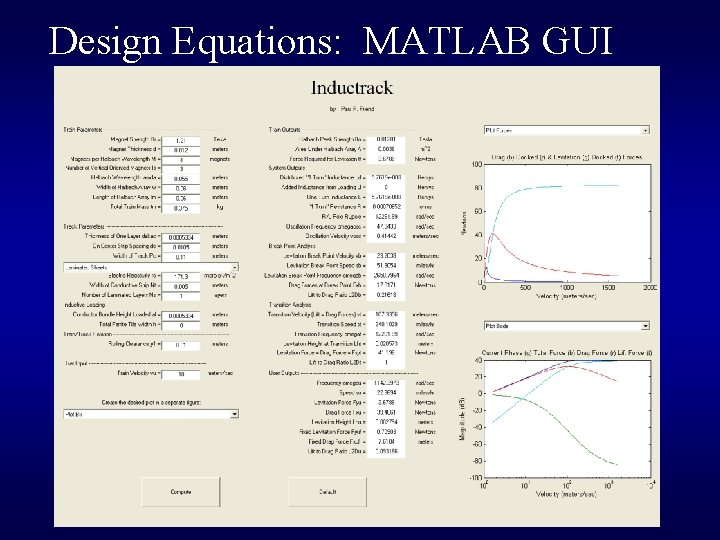Design Equations: MATLAB GUI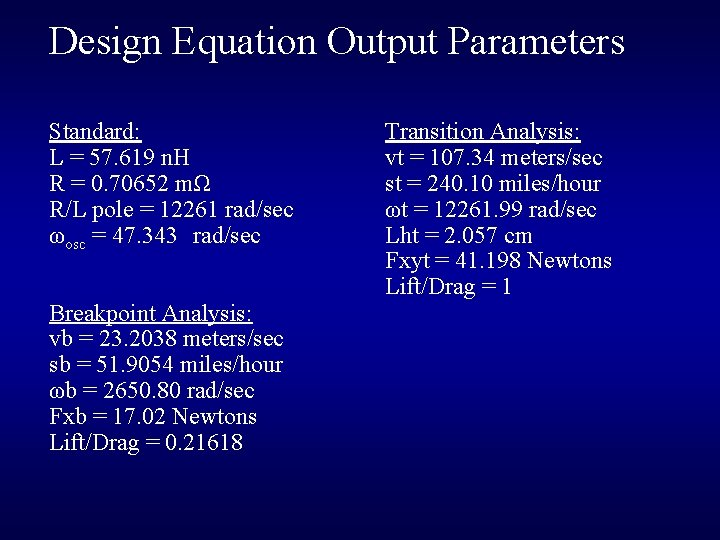Design Equation Output Parameters Standard: L = 57. 619 n. H R = 0. 70652 mΩ R/L pole = 12261 rad/sec ωosc = 47. 343 rad/sec Breakpoint Analysis: vb = 23. 2038 meters/sec sb = 51. 9054 miles/hour ωb = 2650. 80 rad/sec Fxb = 17. 02 Newtons Lift/Drag = 0. 21618 Transition Analysis: vt = 107. 34 meters/sec st = 240. 10 miles/hour ωt = 12261. 99 rad/sec Lht = 2. 057 cm Fxyt = 41. 198 Newtons Lift/Drag = 1Calculated Forces Locked Levitation Transition Velocity Unlocked Levitation Locked Drag Unlocked DragCalculated Forces (Zoomed) Locked Drag Locked Levitation Unlocked Drag Breakpoint VelocityCalculated Forces (Bode) Total Force Drag Force Total Phase Levitation ForceCalculated Levitation Height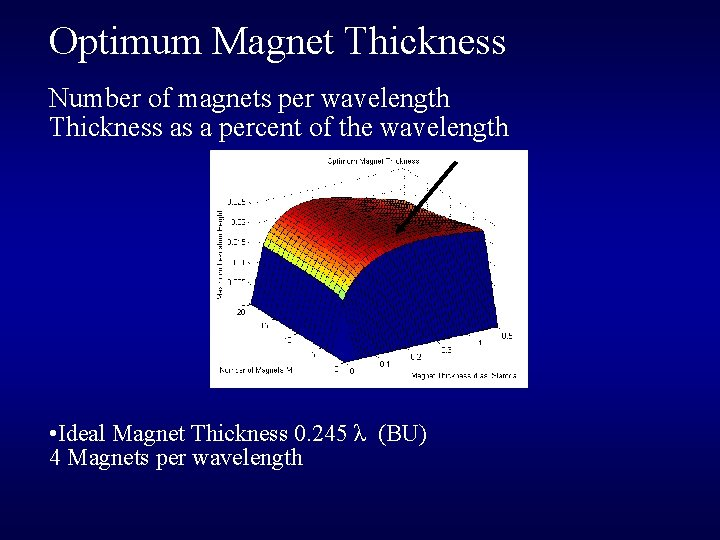Optimum Magnet Thickness Number of magnets per wavelength Thickness as a percent of the wavelength • Ideal Magnet Thickness 0. 245 λ (BU) 4 Magnets per wavelengthPhysical Design Materials Wood and 1/16” Aluminum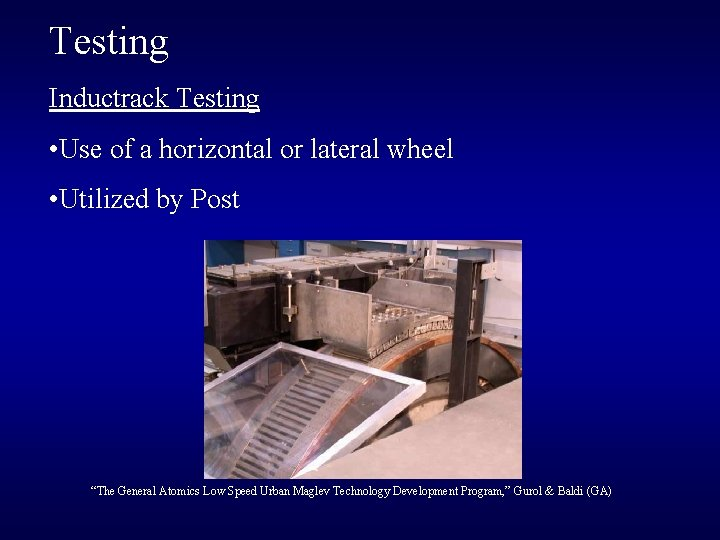Testing Inductrack Testing • Use of a horizontal or lateral wheel • Utilized by Post “The General Atomics Low Speed Urban Maglev Technology Development Program, ” Gurol & Baldi (GA)Test Wheel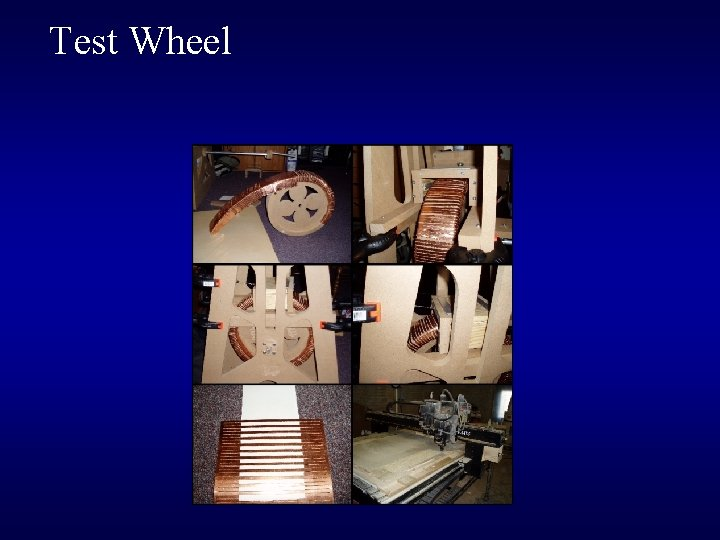Test Wheel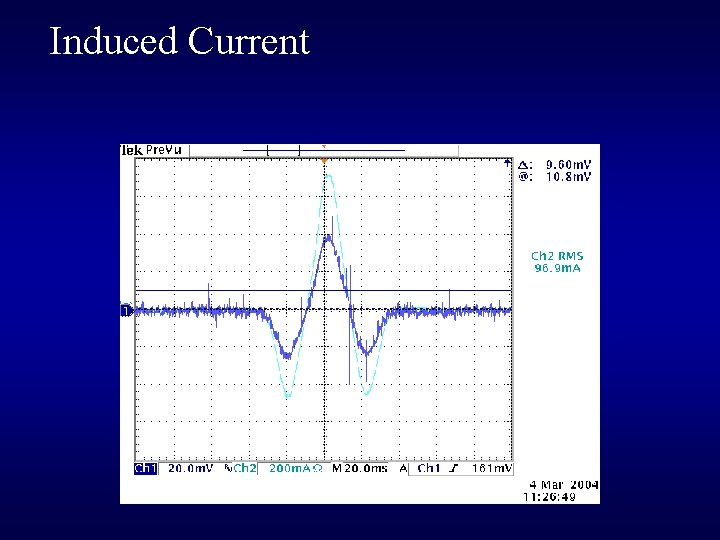Induced Current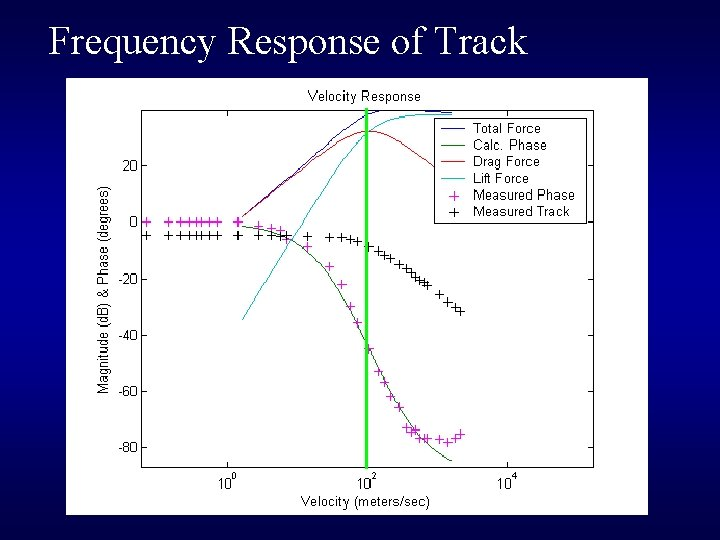Frequency Response of Track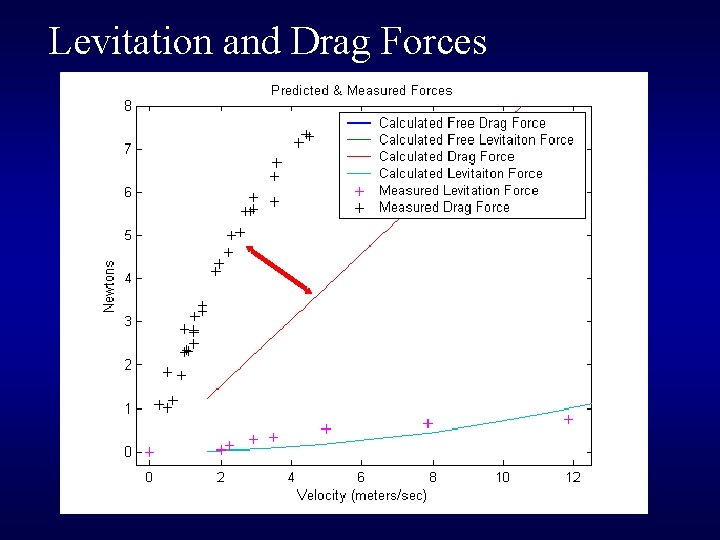Levitation and Drag Forces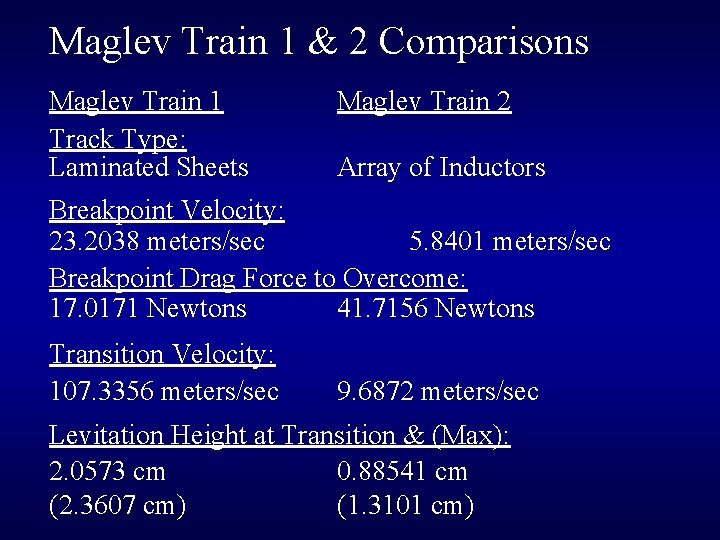Maglev Train 1 & 2 Comparisons Maglev Train 1 Track Type: Laminated Sheets Maglev Train 2 Array of Inductors Breakpoint Velocity: 23. 2038 meters/sec 5. 8401 meters/sec Breakpoint Drag Force to Overcome: 17. 0171 Newtons 41. 7156 Newtons Transition Velocity: 107. 3356 meters/sec 9. 6872 meters/sec Levitation Height at Transition & (Max): 2. 0573 cm 0. 88541 cm (2. 3607 cm) (1. 3101 cm)Maglev Train 1 & 2 Comparisons Maglev Train 1 Maglev Train 2 (Using 5 mm Fixed Height)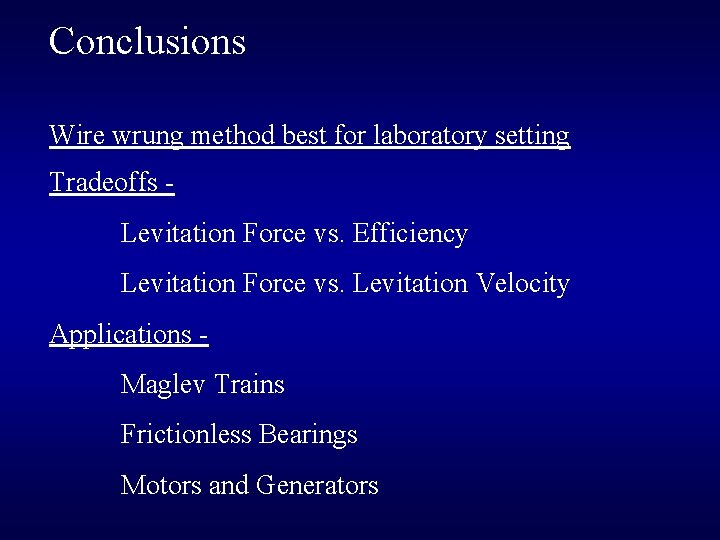Conclusions Wire wrung method best for laboratory setting Tradeoffs Levitation Force vs. Efficiency Levitation Force vs. Levitation Velocity Applications Maglev Trains Frictionless Bearings Motors and GeneratorsTasks Completed and Troubles The Inductrack theory has been understood Magnetic simulations Train has been built Laminated copper track has been built* Testing has occurred* Conclusions have been made (* - trouble)Parts and Equipment 40 - 1/2” Nd. Fe. B, Grade 38 Cubes \$90. 00 40 - 1/4” Nd. Fe. B, Grade 38 Cubes \$14. 40 2 -1/2 Alloy 110 Copper Sheets \$134. 10 Litz-wire Bulks, Copper Sheets, Aluminum Sheets, Wheels, Conductive balls, and Electromagnets Cart/Train non inductive materials and CNC router machine time provided by Midwestern Wood Products Co.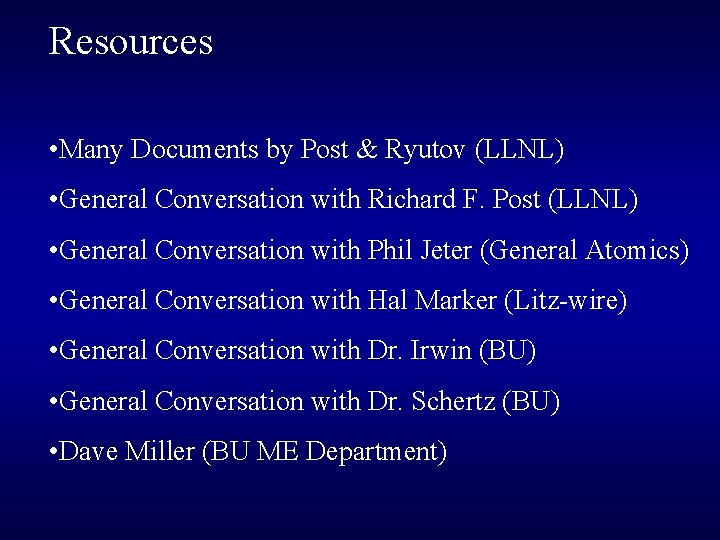Resources • Many Documents by Post & Ryutov (LLNL) • General Conversation with Richard F. Post (LLNL) • General Conversation with Phil Jeter (General Atomics) • General Conversation with Hal Marker (Litz-wire) • General Conversation with Dr. Irwin (BU) • General Conversation with Dr. Schertz (BU) • Dave Miller (BU ME Department)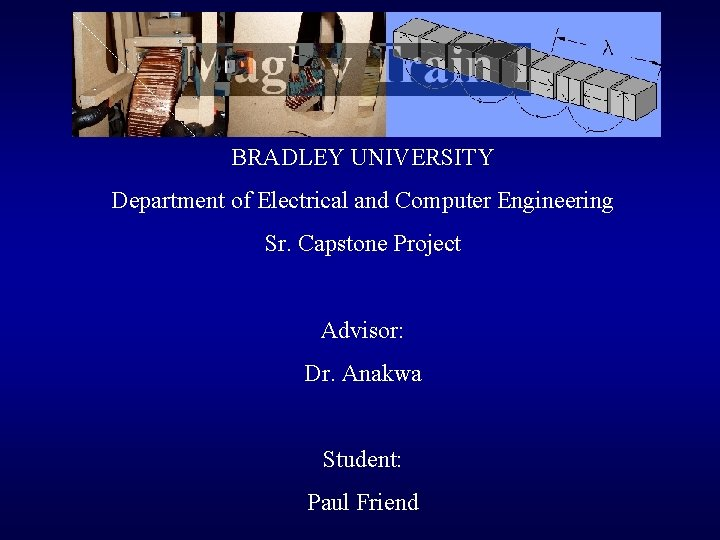BRADLEY UNIVERSITY Department of Electrical and Computer Engineering Sr. Capstone Project Advisor: Dr. Anakwa Student: Paul Friend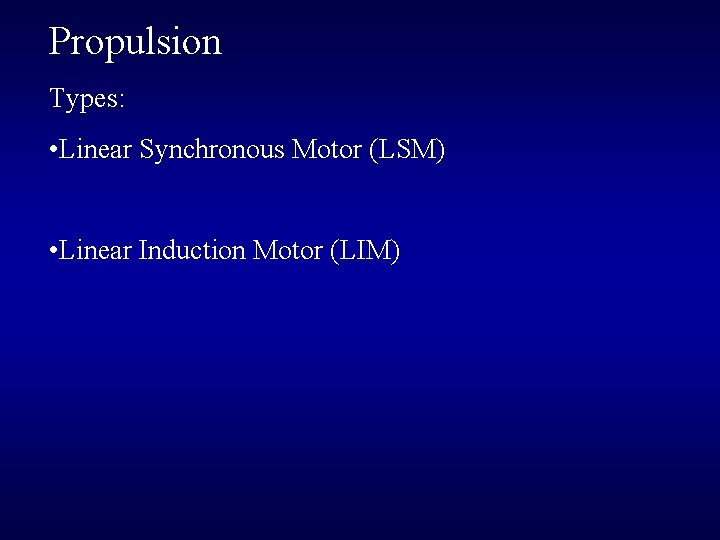Propulsion Types: • Linear Synchronous Motor (LSM) • Linear Induction Motor (LIM)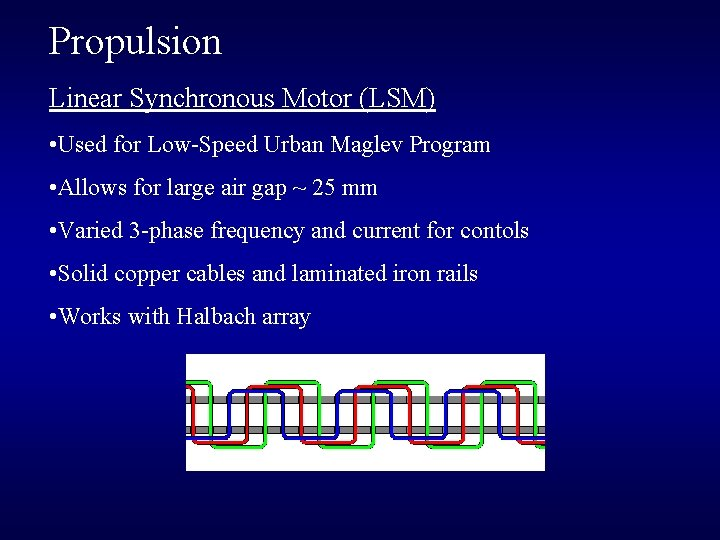Propulsion Linear Synchronous Motor (LSM) • Used for Low-Speed Urban Maglev Program • Allows for large air gap ~ 25 mm • Varied 3 -phase frequency and current for contols • Solid copper cables and laminated iron rails • Works with Halbach array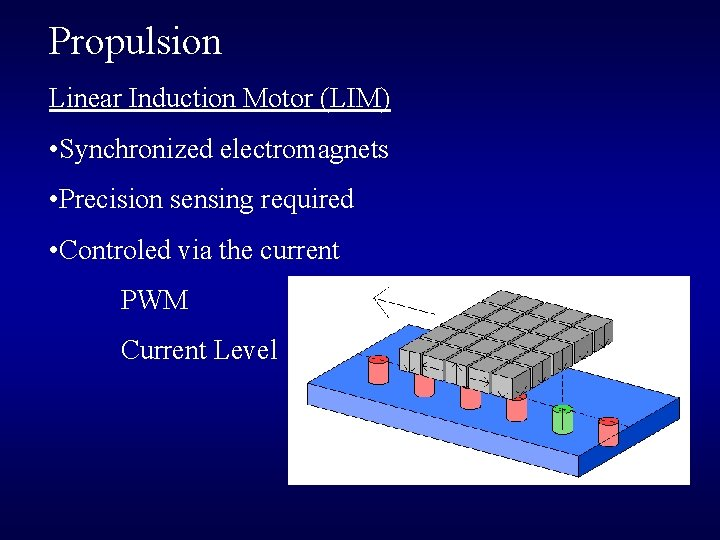Propulsion Linear Induction Motor (LIM) • Synchronized electromagnets • Precision sensing required • Controled via the current PWM Current Level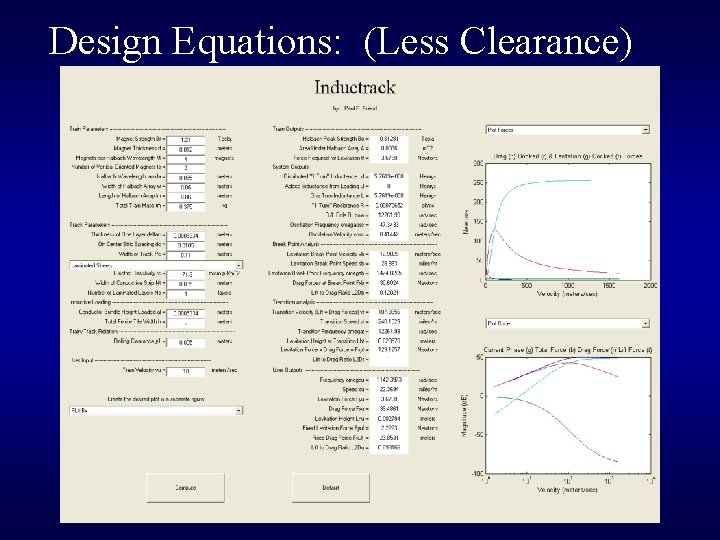Design Equations: (Less Clearance)Design Equations: (Maglev Train 2)